View on GitHub

# Lejon.GitHub.io

### Welcome to Leif Jonssons GitHub Pages.

For fun I keep myself busy implementing some cool algorithms. I also have a Blog (who doesn't?) where I post stuff related to mostly computing. When I get time I'll up some more info here, for now you'll have to settle for my work on the very cool visualization algorithm by Laurens van der Maaten and Geoffrey Hinton called t-SNE. As of 2016-11-03 the implementation supports the Barnes Hut optimization.

Java t-SNE graph (MNIST large)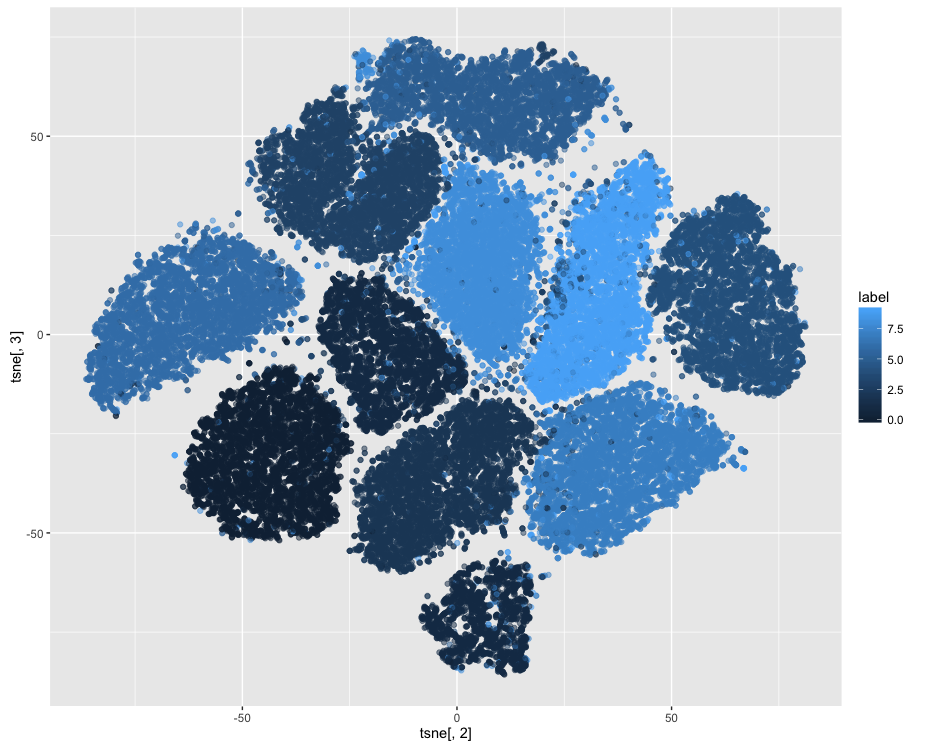t-SNE on 60000 images from MNIST using v2.4.0 of Barnes Hut t-SNE (execution took 18.3 minutes on a 2013 Macbook Pro).

Cheers! -Leif

### t-SNE

Here you can find images generated by my Julia implementation of Laurens van der Maaten's t-SNE and here is the repo. If you prefer Java there is a Java version here.

Julia t-SNE graph (MNIST small)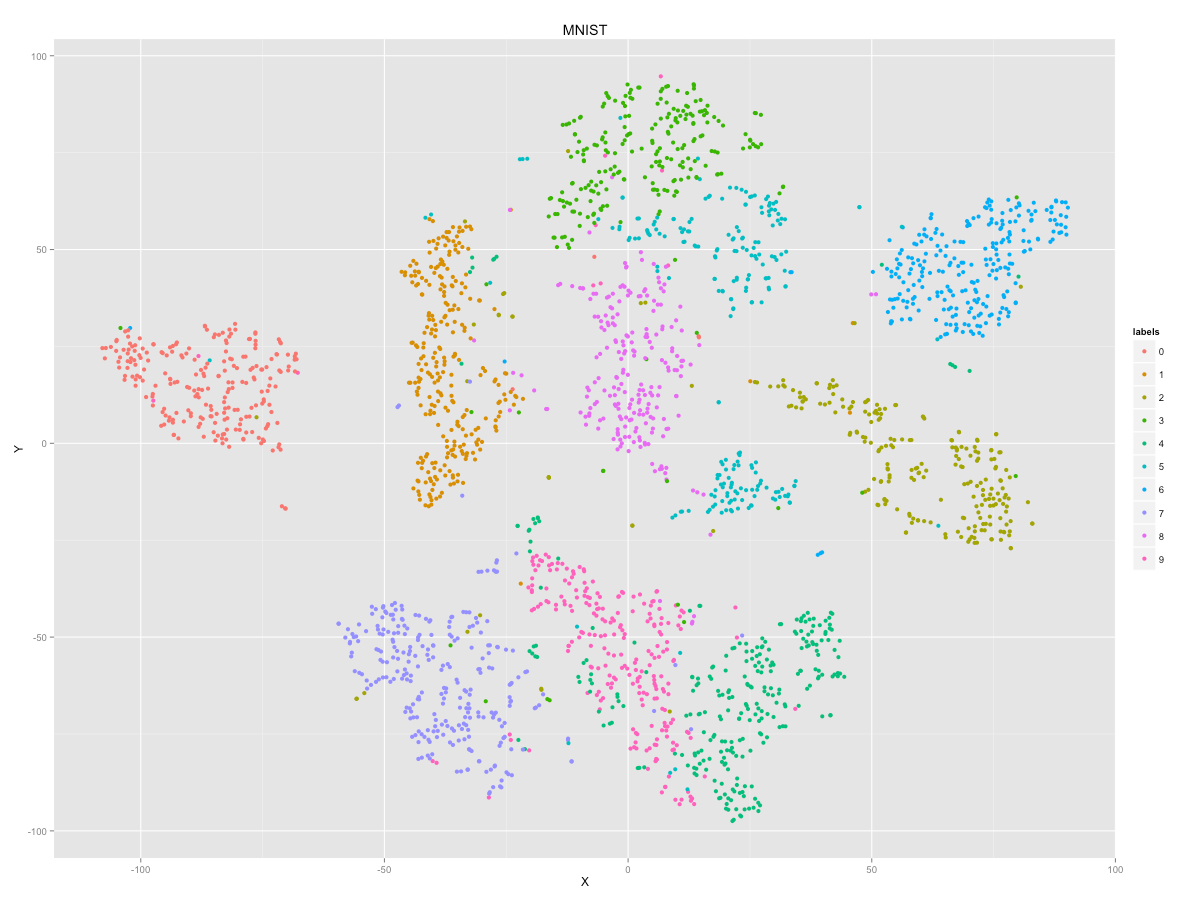Java t-SNE graph (MNIST small)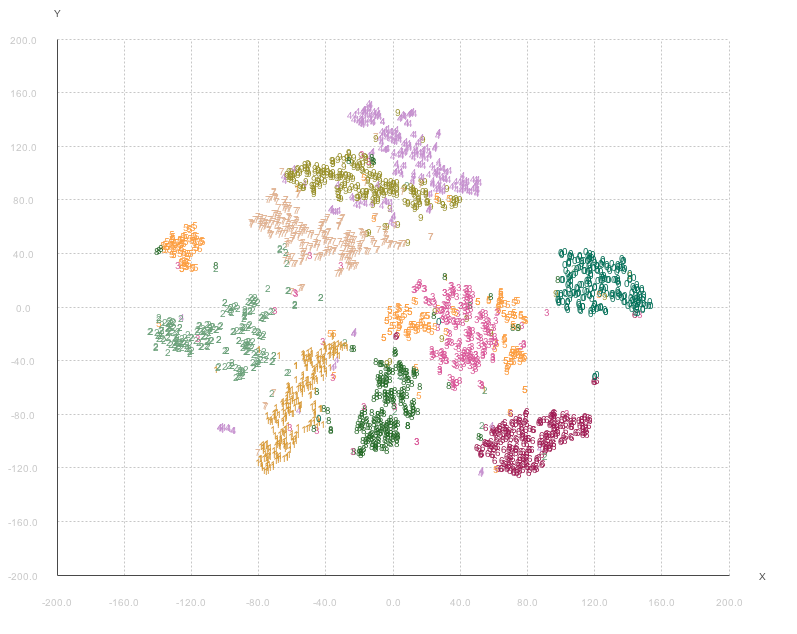### Tips for visualizing with t-SNE

If you have problems getting any results from t-SNE (typically getting the 't-SNE ball'), there are some tricks you can try. Observe that this is not a specific problem with t-SNE, but rather a standard problem in the Machine Learning area.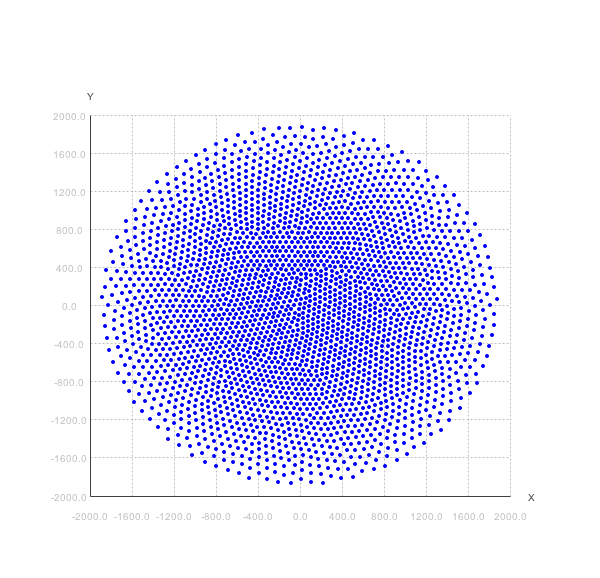The first thing you can try is to center and scale your data. This can be done with the MatrixOps.centerAndScale() method. What this does is subtracting the mean and dividing with the standard deviation of the matrix from each element of the matrix. This is a usual 'trick' to get the data to be on the same scale.

``````double[][] matrix = your data here
matrix = MatrixOps.centerAndScale(matrix);
``````

Another trick you can try, and this is typically if you have counts in the matrix and lots of zero counts (so called zero inflated data) is to take the log of all the values in the matrix. Now, you have to take care that the zeros are preserved when doing this and the log operation will not typically do this for you. The t-SNE package have a helper method for this called MatrixOps.log(matrix, true); where the 'true' means, keep zeros as zero and not -Infinity.

``````double[][] matrix = your data here
matrix = MatrixOps.log(matrix, true);
``````

Note: It is important that if you need to do both log and centerAndScale, you need to do the log operation first!

``````double[][] matrix = your data here
matrix = MatrixOps.log(matrix, true);
matrix = MatrixOps.centerAndScale(matrix);
``````

### More t-SNE Examples

Here is an example of running t-SNE on the 20-newsgroups dataset with our Parallel LDA implementation described in our article Parallelizing LDA using Partially Collapsed Gibbs Sampling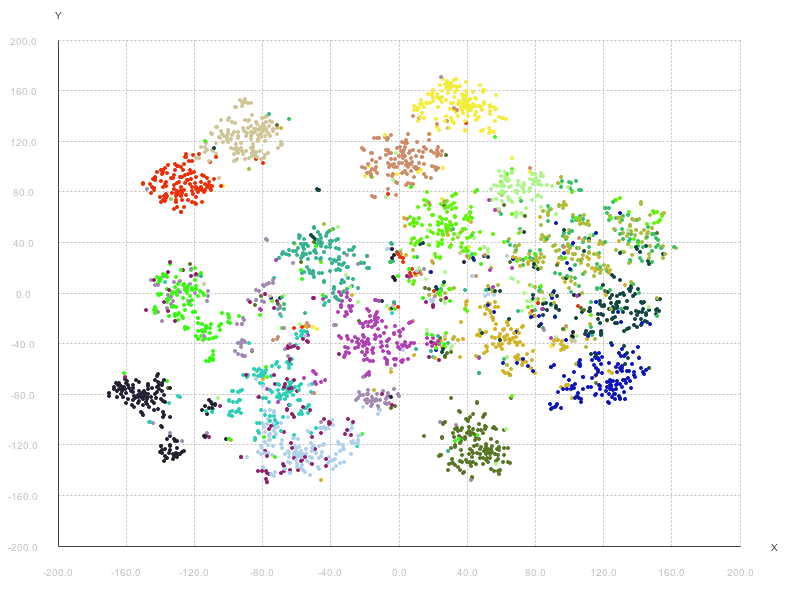### That's all!

For now...

Having trouble with Pages? Check out the documentation at https://help.github.com/pages or contact support@github.com and we’ll help you sort it out.